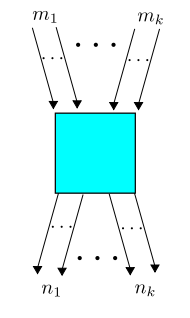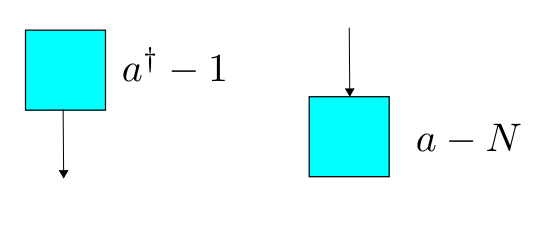# The Azimuth Project Blog - network theory (Biamonte guest posts)

This page contains material on stochastic Petri nets and chemical reaction networks written by Jacob Biamonte. It may go into one or more blog posts. To discuss this page as it is being written, go to the Azimuth Forum. See also Blog - network theory (amoeba post) and Blog - network theory (examples).

## Chemical reaction networks

This paper:

• D. F. Anderson, G. Craciun and T.G. Kurtz, Product-form stationary distributions for deficiency zero chemical reaction networks, arXiv:0803.3042.

proves that for a large class of stochastic Petri nets, there exist equilibrium solutions of the master equation where the number of things in each state is distributed according to a Poisson distribution. Even more remarkably, these probability distributions are independent, so that knowing how many things are in one state tells you nothing about how many are in another. A nice quote from the paper is

“The surprising aspect of the deficiency zero theorem is that the assumptions of the theorem are completely related to the network of the system whereas the conclusions of the theorem are related to the dynamical properties of the system.”

For a stochastic Petri net to have these marvellous properties, it suffices that it have ‘deficiency zero’ and obey the ‘complex balancing condition’. These conditions are widely used in studying equilibrium solutions for the rate equation of a chemical reaction network. A good introductory treatment to these conditions is:

• Jeremy Gunawardena, Chemical reaction network theory for in-silico biologists.

However, Anderson noted that the complex balancing condition is also equivalent to the ‘nonlinear traffic equations’ in queuing theory, as explained here:

• F. Kelly, Reversibility and Stochastic Networks, Wiley, New York, 1979.

• J. Mairesse and H.-T. Nguyen, Deficiency zero Petri nets and product form, arXiv:0905.3158.

So, we see that these ideas show up in quite different applications. Section 6 of the following paper:

• Eduardo Sontag and Doron Zeilberger, Symbolic computational approach to a problem involving multivariate Poisson distributions, Advances in Applied Mathematics 44 (2010), 359-377.

quickly reviews this material and gives examples. Here we will study some examples using quantum field theory techniques.

## From chemical reaction networks to Petri nets

Chemical reaction networks are a well used formalism to describe the differential equations emerging from chemical reaction modelling. This formalism turns out to be just as descriptive as the Petri net formalism, and each of these networks can be translated into the other. Our focus here will be to translate chemical reaction networks into Petri nets, so we can apply methods from the Petri net field theory we talked about before.

### Chemical reaction networks

In chemical reactions, we consider chemical species as the inputs, intermittent and resulting chemicals from a given reaction.

Definition. (Chemical reaction network [Anderson et al.]). Let $S= \{S_i\}$, $C=\{v_k\}$, and $R=\{v_k\rightarrow v_k'\}$ denote the sets of species, complexes, and reactions, respectively. The triple $\{S, C, R\}$ is called a chemical reaction network.

# Stability of solutions

A stable equilibrium is an equilibrium solution such that all solutions with sufficiently close initial data converge to that equilibrium solution as $t \to + \infty$.

## Baez-Brain Dump, star date: 4-12-2011

Today I ran into a massive Baez quasar, which resulted in my sensors being overloaded with 7 pages of raw data.

### Stochastic Petri Net

States: $S=\{1,...,k\}$

Transitions: $T$. We consider $\tau \in T$ such that

$\tau$ has inputs $m(\tau) = (m_1(\tau), ..., m_k(\tau))\in N^k$

$\tau$ has outputs $n(\tau) = (n_1(\tau), ..., n_k(\tau))\in N^k$

and rate constant $r(\tau)\in R$

### Rate equation

Starting from a petri net, we arrive at a rate equation.

Let $x_i(t)$ be the amount of concentration of the ith state $(i=1,...,k)$

$\frac{d x_i(t)}{dt} = \sum_{\tau\in T} r(\tau) x_1^{m_1(\tau)}(t) \cdots x_k^{m_k(\tau)}(t)(n_i(\tau)-m_i(\tau))$

Theorem 1. (Feinberg). If the Petri net is weakly reversible and has deficiency 0 then $\exists x_i\in R^k$ such that, RHS = 0.

#### Example

[PIC Here]

$k=1$ $T=\{1,2\}$ rate constants $r_1$ and $r_2$.

$\frac{dx_1}{dt} = -r_1 x_1^2 + r_2x_1$

$\exists x_1 \in R$ such that,

$-r_1x_1^2+r_2x_1=0$

### Master equation

We also arrive at a master equation.

Now say we have a probability,

$\psi_{i_1...i_k}$ of having $i_1$ things in state 1, $i_k$ things in state $k$ etc. We write

$\psi = \sum \psi_{i_1...i_k}z_1^{i_1}\cdots z_k^{i_k}$

and $a_i^\dagger \psi = z_i \psi$, $a_i\psi = \frac{\partial}{\partial z_i} \psi$ then the master equation says

$\frac{d}{dt}\psi = H\psi$

Each transition corresponds to an operator. There is a term $a_1^{\dagger n_1}\cdots a_k^{\dagger n_1} a_1^{m_1}\cdots a_k^{m_k}$but we also have to subtract off an additional factor, given by the number operator. This results in

$a_1^{\dagger n_1}\cdots a_k^{\dagger n_1} a_1^{m_1}\cdots a_k^{m_k} - N_1^{\underline{m}_1}\cdots N_k^{\underline{m}_k}$

where $N_i = a^\dagger_i a_i$ and

$N_i^{\underline m_i} = N_i(N_i -1)(N_i-2)\cdots (N_i-m_i+1)$Explain falling towers here.

In general

$H = \sum_{\tau \in T} r(\tau) (a_1^{\dagger n_1(\tau)}\cdots a_k^{\dagger n_k(\tau)}a_1^{m_1(\tau)}\cdots a_k^{m_k(\tau)}- N_1^{\underline m_1}\cdots N_k^{\underline m_k})$

Solutions.

To solve $H\psi = 0$, try

$\psi = \prod_{i=1}^k \sum_{n_i = 0}^\infty \frac{\alpha_i^{n_i}}{n_i!} z_i^{n_i} = \prod_{i=1}^k e^{\alpha_iz_i}$

### Digression on coherent states

We make heavy use of coherent states in this project.

We let $a^\dagger$ ($a$) be creation (destruction) operators and define the number operator as $N:= a^\dagger a$. Then

• $[N, a^\dagger]=a^\dagger$, $[a, N]=a$ and $[a, a^\dagger] = 1$

#### Markov processes

We could be more general, but let’s keep things simple and suppose we a physical system with some set of states $X$. Then a Markov process gives a rule for computing, at any time $t \ge 0$, the probability of hopping from any state $j \in X$ to any state $i \in X$. This rule gives us a matrix $U(t)$, but this matrix needs to obey some properties. Tersely put:

Definition. A Markov process consists of a set $X$ and a strongly continuous one-parameter group of stochastic operators on $L^1(X)$.

But we should remind you what all these buzzwords mean! First, we’re using ‘stochastic state’ as an overly fancy synonym for ‘probability distribution’:

Definition. A stochastic state is a function $\psi: X \to [0,\infty)$ with

$\sum_{i \in X} \psi_i = 1$

We think of stochastic states as living in the vector space

$L^1(X) = \{ \psi: X \to \mathbb{R} \; : \; \int_X |\psi(x)| \, d x < \infty \}$

so we say:

Definition. An operator $U : L^1(X) \to L^1(X)$ is stochastic if it maps stochastic states to stochastic states.

We want to describe time evolution in our Markov process using a ‘one-parameter semigroup’ of stochastic operators:

Definition. A one-parameter semigroup of stochastic operators consists of a stochastic operator $U(t)$ for each time $t \ge 0$, obeying

$U(0) = I$

and

$U(t) U(s) = U(t+s)$

But it will be ridiculous if our semigroup isn’t continuous in a certain sense:

Definition. A one-parameter semigroup of stochastic operators $U(t)$ is continuous if

$t_i \to t \quad \implies \quad U(t_i) \psi \to U(t) \psi$

where convergence is in the usual norm topology on $L^1(X)$….

an infinitesimal stochastic matrix $H_{i j}$, where $i, j \in X$.

We’ll remind you what ‘infinitesimal stochastic’ means in a second, but the point is that for $i \ne j$, the matrix element $H_{i j}$ describes the probability per unit time of hopping from the state $j \in X$ to the state $i \in X$. So, if $\psi_i(t)$ is the probability of our system being in the state $i$ at time $t$, we want this equation to hold…

#### Example of Noether's theorem

Fragmentary stuff:

And in fact, we can think of the total number of things as an operator on our space of formal power series:

$N = N_1 + N_2$

where $N_1$ and $N_2$ are the number operators we’ve seen so often before:

$N_i = a_i^\dagger a_i = z_i \frac{\partial}{\partial z_i}$

What exactly is the relation between $N$ and the total number of things? Part of the answer is this: the expected total number of things in any state $\Phi$ is given by

$\sum N \Phi$

Let’s see why!

First of all, what does this expression even mean? The funny ‘sum’ notation here was introduced in Part 6, but now I’m using it for power series in two variables instead of one. Namely, for any power series

$\Phi = \sum_{n_1, n_2 = 0}^\infty \phi_{n_1, n_2} z_1^{n_1} z_2^{n_2}$

we define

$\sum \Phi = \sum_{n_1, n_2 = 0}^\infty \phi_{n_1, n_2}$

Thus, $\sum \Phi = 1$ whenever $\Phi$ is a state, since probabilities must sum to 1. But we also have

$\begin{array}{ccl} N \Phi &=& \sum_{n_1, n_2 = 0}^\infty \phi_{n_1, n_2} (z_1 \frac{\partial}{\partial z_1} + z_2 \frac{\partial}{\partial z_2}) z_1^{n_1} z_2^{n_2} \\ &=& \sum_{n_1, n_2 = 0}^\infty (n_1 + n_2) \phi_{n_1, n_2} z_1^{n_1} z_2^{n_2} \end{array}$

so that

$\sum N \phi = \sum_{n_1, n_2 = 0}^\infty (n_1 + n_2) \phi_{n_1, n_2}$

And this is exactly the expected value of the total number of things.

So, for this and other reasons, we can think of the operator $N$ an ‘observable’ that counts the total number of things. Now, in quantum mechanics Noether’s theorem tells us that an observable is a conserved quantity—it doesn’t change with time—if it commutes with the Hamiltonian. So you should suspect that

$[H,N] = 0$

where the commutator $[H,N]$ is defined to be $H N - N H$.

Puzzle 2. Is $[H,N] = 0$?

If this is true, it should follow that $H$ will commute with any function of the operator $N$, for example the function

$\delta(N - n)$

where $\delta$ is the Kronecker delta, which equals 1 at the origin and is zero elsewhere. This operator should be a projection operator, and it should project to an eigenspace of $N$, say

$\{ \Phi : N \Phi = n \Phi \}$

and get a new equilibrium state, say $\Phi_n$:

$H \Psi_n = 0$

category: blog